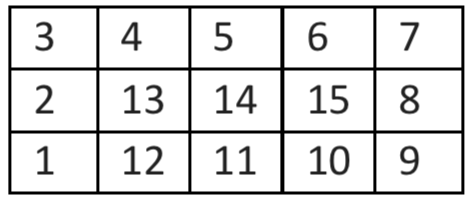# Problem

In a theater, Seats are arranged in the N by M grid. The number of each seat is indicated by the coordinates of the corresponding grid. For example, when N = 3 and M = 5, the seats are like below.Many people stand in waiting lines to enter this venue. The waiting people receive the waiting list in the order 1, 2, 3, 4, ... from the front. We start with (1, 1) seats for people with a waiting number, turn clockwise and assign the audience to the empty seats in order like below.Visual aid:If N and M are given, a program should be created to find the seat number (x, y) to be assigned to the audience whose waiting sequence is K. If there is no seat for K, the program returns 0.

T = number of test case

Input

T

N M K

N M K

Output

x y

x y

Example

Input

3

6 7 11

6 7 87

100 100 3000

Output

66 0

9 64

# Solution

``````import java.util.Scanner;

public class Theater {

private static void printMap(int[][] map) {
for (int[] a : map) {
for (int b : a) {
System.out.printf("%-5d", b);
}
System.out.println();
}
System.out.println();
}

public static void main(String[] args) {
String i = "3\n" +
"7 6 31\n" +
"7 6 87\n" +
"100 100 3000\n";

Scanner s = new Scanner(i);

int T = s.nextInt();
for (int t = 0; t < T; t++) {
int N = s.nextInt();
int M = s.nextInt();
int K = s.nextInt();

if (K > N * M) {
// dont fit
System.out.println(0);
continue;
}

int[][] mat = new int[M][N];

int u = 0, r = 0, d = 0, l = 0;
int x = 0, y = M;
int dir = 0; // 0 = up, 1 = right, 2 = down, 3 = left

for (int move = 1; move < K + 1; move++) {
if (dir == 0) {
if (y - 1 - u == 0) {
dir = 1;
l++;
}
mat[--y][x] = move;
} else if (dir == 1) {
if ((x + r) + 2 == N) {
dir = 2;
u++;
}
mat[y][++x] = move;
} else if (dir == 2) {
if ((y + 2) + d == M) {
dir = 3;
r++;
}

mat[++y][x] = move;
} else if (dir == 3) {
if (x - l == 1) {
dir = 0;
d++;
}
mat[y][--x] = move;
}

}
printMap(mat);
System.out.println((x + 1) + " " + (M - y));
}
}
}
``````

Sorry, limited comments! That's up to you.

Disclaimer: Don't copy paste the code if you're going to submit! Change it to be yours! I am not responsible if you get in any trouble. This post is only meant for me to come back to later when practicing. It is not in my control to if anyone comes and copies it. Have a good day.